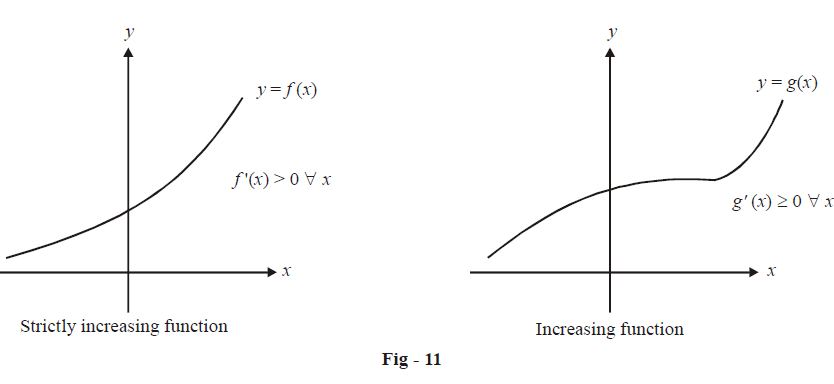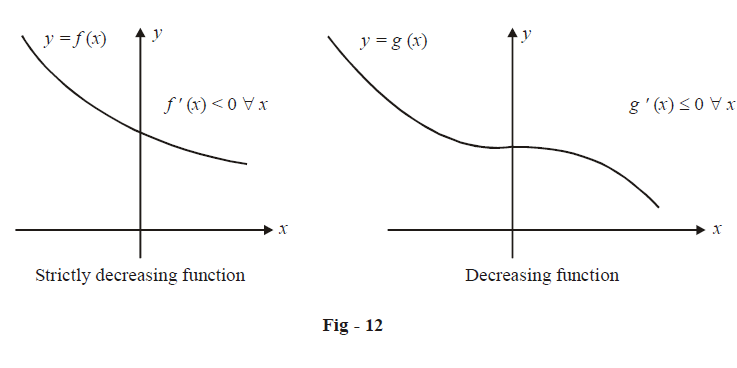# Analysing Monotonicity

Go back to  'Applications of Derivatives'

Let us now deduce the condition(s) on the derivative of a function f(x) which determines whether f(x) is increasing/decreasing on a given interval. We are assuming that f(x) is everywhere differentiable.The function on the left side, $$y = f\left( x \right),$$ is a strictly increasing function. Notice that the slope of the tangent drawn at any point on this curve is always positive. Hence, a sufficient condition for f(x) to be strictly increasing on a given domain D is

$f'\left( x \right) > 0\,\,\,\,\,\forall x \in D$

Later on, we will see that this is not a necessary condition for a function to be strictly increasing.

In Fig-11, the function on the right side, $$y = g\left( x \right)$$  is not strictly increasing though it is increasing. Notice that  $$g'\left( x \right) > 0$$ or  $$g'\left( x \right) = 0\,\,\,{\text{for }}\;\forall x.$$ $$g'\left( x \right)$$ is never negative. Hence, a sufficient condition for g(x) to be increasing on a given domain D is

$g'\left( x \right) \ge 0\,\,\,{\rm{ }}\forall x \in D$

*     Note that for these condition on the derivatives to be applied, the function must be differentiable in the given domain. However, these conditions will hold good even if the function is non differentiable, but only at a finite number (or infinitely countable number) of points. For eg, $$f\left( x \right) = \left[ x \right] + \sqrt {\left\{ x \right\}}$$  is strictly increasing on  $$\mathbb{R}.$$ However, f(x) is non-differentiable at all integers (a countable set).

*     A function must be continuous for these conditions to be applied. Consider $$y = \left\{ x \right\}.$$  This is non-differentiable (due to discontinuities) at all integers. At all other points,  \begin{align}y' = 1 > 0.\end{align} However, we know that  $$y = \left\{ x \right\}$$ is not strictly increasing.

Similarly, \begin{align} y = \frac{1}{x} \end{align}  is non-differentiable (and non-continuous) at x = 0. At all other points, \begin{align}y' = \frac{{ - 1}}{{{x^2}}} < 0\end{align}  so that y should be strictly decreasing on $$\mathbb{R}\backslash \left\{ 0 \right\}$$ However, it is not strictly decreasing on $$\mathbb{R}\backslash \left\{ 0 \right\}$$ although it is strictly decreasing in the separate intervals $$\left( { - \infty ,0} \right)$$ and $$\left( {\infty ,0} \right).$$

Therefore, we see that discontinuous functions cannot be subjected to the derivative condition even though they may be discontinuous only at a finite (or infinitely countable) number of points.

Now consider f(x) and g(x) in Fig 12Extending the previous case, we get the conditions for a (strictly) decreasing function:

 Strictly decreasing : $$f'\left( x \right) < 0\;\;\;\;\;\;\; \forall x \in D$$ Decreasing : $$f'\left( x \right) \le 0\;\;\;\;\;\;\; \forall x \in D$$

The remarks made for the increasing case hold true here also.

Before concluding this section, here are some other facts worth paying attention to:

(a)   If f(x) is strictly increasing, then$${f^{ - 1}}(x)$$ is also strictly increasing. Similarly, if f(x) is strictly decreasing then $${f^{ - 1}}(x)$$ is also strictly decreasing.

(b)   If f(x) and g(x) have the same monotonicity (both increasing or decreasing) on [a, b], then  and  are monotonically increasing on [a, b].

(c)   If f(x) and g(x) have opposite monotonicity on [a, b], then $$f\left( {g\left( x \right)} \right)$$  and$$g\left( {f\left( x \right)} \right)$$ are strictly decreasing on [a, b]

(d)   The inverse of a continuous function is continuous

(e)   If $$f'\left( x \right) > 0\,\,\,\forall x \in \left( {a,\,\,b} \right)$$  except for a finite (or an infinitely countable) number of points where $$f'\left( x \right) = 0,\,\,f\left( x \right)$$  is still strictly increasing on (a, b). This is why we said earlier that  $$f'\left( x \right) > 0\,\,\,\,\,\,\forall x \in D$$  is not a necessary condition for strict increase. For example, in a later example we will consider the graph of the function $$f\left( x \right) = x + \cos x.$$  We will see that $$f'\left( x \right) = 1 - \sin x$$  is not always positive \begin{align}\left( {{\text{at}}\,x = 2n\pi + \frac{\pi }{2},n \in \mathbb{Z},\,f'\left( x \right) = 0} \right)\end{align} ; even then, f(x) increases strictly, because the points at which f'(x) = 0 are countable

(f)   Similarly if $$f'\left( x \right) < ,\,\,\,0\forall x \in \left( {a,\,\,b} \right)$$  except for a finite (or an infinitely countable) number of points     where $$f'\left( x \right) = 0,\,\,f\left( x \right)$$  is still strictly decreasing on (a, b)